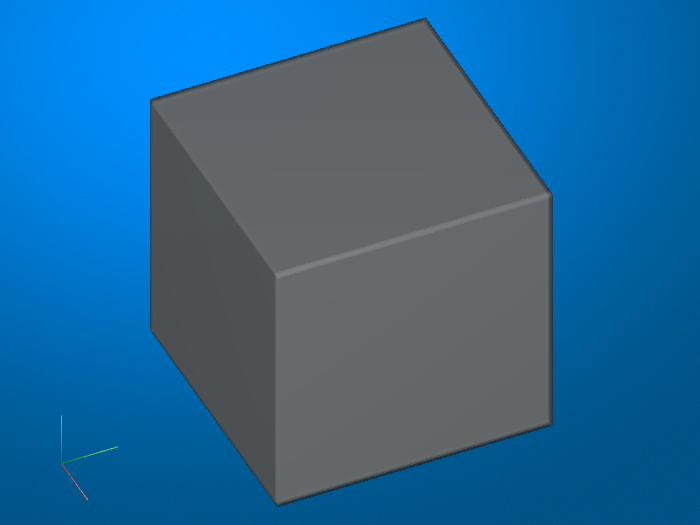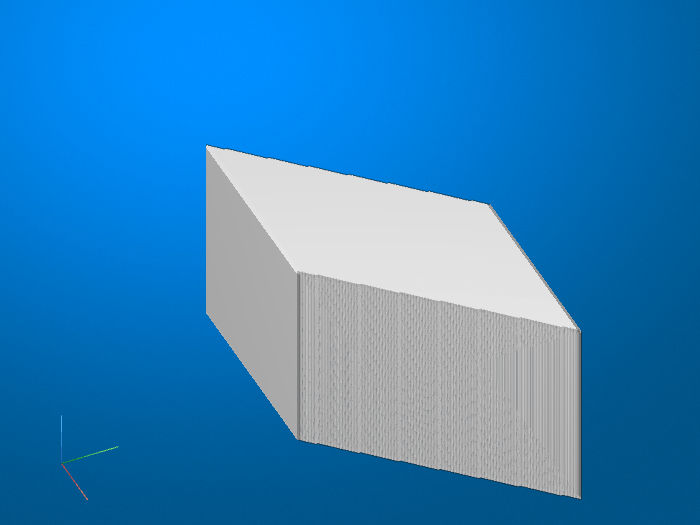# randomAffine3d

Create randomized 3-D affine transformation

Since R2019b

## Syntax

``tform = randomAffine3d``
``tform = randomAffine3d(Name,Value)``

## Description

````tform = randomAffine3d` creates an `affinetform3d` object that performs an identity transformation.```

example

````tform = randomAffine3d(Name,Value)` specifies the type of affine transformation using name-value arguments.```

## Examples

collapse all

Create a sample volume.

`volumeCube = 0.5*ones(100,100,100);`

Create a 3-D viewer to control the appearance of the scene. Set the camera position of the scene to improve the visibility of the volume.

`viewer = viewer3d(CameraPosition=[700 -250 650],CameraTarget=[50 50 50]);`

Display the volume in the scene.

`volshow(volumeCube,Parent=viewer);`Create a 3-D affine transformation that shears 3-D volumes. The `randomAffine3d` function picks a shear amount randomly from a continuous uniform distribution within the interval [40, 60] degrees. `randomAffine3d` picks a random shear direction aligned with the x-, y-, or z-axis.

```tform1 = randomAffine3d(Shear=[40 60]); J1 = imwarp(volumeCube,tform1);```

Display the sheared volume in a new scene with the same camera position.

```viewer1 = viewer3d(CameraPosition=[700 -250 650],CameraTarget=[50 50 50]); volshow(J1,Parent=viewer1);```To shear a volume by a different randomly selected amount, create a new 3-D affine transformation. Note the difference in the shear direction.

```tform2 = randomAffine3d(Shear=[40 60]); J2 = imwarp(volumeCube,tform2);```

Display the sheared volume in a new scene with the same camera position.

```viewer2 = viewer3d(CameraPosition=[700 -250 650],CameraTarget=[50 50 50]); volshow(J2,Parent=viewer2);```## Input Arguments

collapse all

### Name-Value Arguments

Specify optional pairs of arguments as `Name1=Value1,...,NameN=ValueN`, where `Name` is the argument name and `Value` is the corresponding value. Name-value arguments must appear after other arguments, but the order of the pairs does not matter.

Example: `tform = randomAffine3d(XReflection=true)`

Before R2021a, use commas to separate each name and value, and enclose `Name` in quotes.

Example: `tform = randomAffine3d("XReflection",true)`

Random horizontal reflection, specified as `false` or `true`. When `XReflection` is `true` (`1`), the transformation `tform` reflects images horizontally with 50% probability. By default, the transformation does not reflect images in the horizontal direction.

Random vertical reflection, specified as `false` or `true`. When `YReflection` is `true` (`1`), the transformation `tform` reflects images vertically with 50% probability. By default, the transformation does not reflect images in the vertical direction.

Random reflection along the depth direction, specified as `false` or `true`. When `ZReflection` is `true` (`1`), the transformation `tform` reflects images along the depth direction with 50% probability. By default, the transformation does not reflect images in the depth direction.

Range of rotation applied to the input image, specified as one of the following. Rotation is measured in degrees.

• A 2-element numeric vector. The second element must be larger than or equal to the first element. `randomAffine3d` picks a rotation angle randomly from a continuous uniform distribution within the specified interval. `randomAffine3d` selects a random axis of rotation from the unit sphere.

• A function handle of the form

`[rotationAxis,theta] = selectRotation`
The function `selectRotation` must accept no input arguments. The function must return two output arguments: `rotationAxis`, a 3-element vector defining the axis of rotation, and `theta`, a rotation angle in degrees.

Use a function handle to pick rotation angles from a disjoint interval or using a nonuniform probability distribution. You can also use a function handle to specify an axis of rotation. For more information about function handles, see Create Function Handle.

By default, the transformation `tform` does not rotate images.

Example: `[-45 45]`

Range of uniform (isotropic) scaling applied to the input image, specified as one of the following.

• A 2-element numeric vector. The second element must be larger than or equal to the first element. The scale factor is picked randomly from a continuous uniform distribution within the specified interval.

• A function handle. The function must accept no input arguments and return the scale factor as a numeric scalar. Use a function handle to pick scale factors from a disjoint interval or using a nonuniform probability distribution. For more information about function handles, see Create Function Handle.

By default, the transformation `tform` does not scale images.

Example: `[0.5 4]`

Range of shear applied to the input image, specified as one of the following. Shear is measured as an angle in degrees, and is in the range (–90, 90).

• A 2-element numeric vector. The second element must be larger than or equal to the first element. The shear angle is picked randomly from a continuous uniform distribution within the specified interval. `randomAffine3d` applies shear with uniform randomness to one of the principle x-, y-, and z-directions with respect to one of the two possible orthogonal directions.

• A function handle. The function must accept no input arguments and return the shear angle as a numeric scalar. Use a function handle to pick a shear angle from a disjoint interval or using a nonuniform probability distribution. For more information about function handles, see Create Function Handle.

By default, the transformation `tform` does not shear images in the horizontal direction.

Example: `[0 45]`

Range of horizontal translation applied to the input image, specified as one of the following. Translation distance is measured in pixels.

• A 2-element numeric vector. The second element must be larger than or equal to the first element. The translation distance is picked randomly from a continuous uniform distribution within the specified interval.

• A function handle. The function must accept no input arguments and return the translation distance as a numeric scalar. Use a function handle to pick a translation distance from a disjoint interval or using a nonuniform probability distribution. For more information about function handles, see Create Function Handle.

By default, the transformation `tform` does not translate images in the horizontal direction.

Example: `[-5 5]`

Range of vertical translation applied to the input image, specified as one of the following. Translation distance is measured in pixels.

• A 2-element numeric vector. The second element must be larger than or equal to the first element. The translation distance is picked randomly from a continuous uniform distribution within the specified interval.

• A function handle. The function must accept no input arguments and return the translation distance as a numeric scalar. Use a function handle to pick a translation distance from a disjoint interval or using a nonuniform probability distribution. For more information about function handles, see Create Function Handle.

By default, the transformation `tform` does not translate images in the vertical direction.

Example: `[-5 5]`

Range of translation along the depth direction applied to the input image, specified as one of the following. Translation distance is measured in pixels.

• A 2-element numeric vector. The second element must be larger than or equal to the first element. The translation distance is picked randomly from a continuous uniform distribution within the specified interval.

• A function handle. The function must accept no input arguments and return the translation distance as a numeric scalar. Use a function handle to pick a translation distance from a disjoint interval or using a nonuniform probability distribution. For more information about function handles, see Create Function Handle.

By default, the transformation `tform` does not translate images in the depth direction.

Example: `[-5 5]`

## Output Arguments

collapse all

Affine transformation, returned as an `affinetform3d` object.

## Version History

Introduced in R2019b

expand all